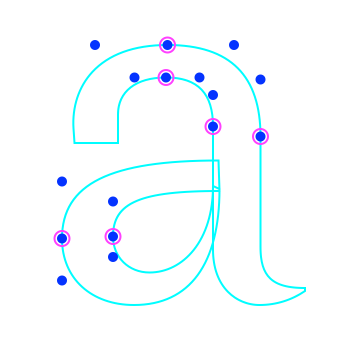# SOLVEDEasier way of getting all selected contour points

• Hellow!

In RF if the user selects the on-curve point, the off-curve is implicitly selected too, but is not returned in `c.selectedPoints`. One workaround is to convert the RPoint to RBPoint which is throwing traceback sometimes. `c.selectedBPoints` doesn't return the off curve if I've only selected the off curve. Is there an ultimate way to get all the selected points (implicit/explicit) without jumping through hoops?

Thanks

• the `try/except` is not really needed, I just forgot to wrap the next point index in parenthesis before performing the modulo division.

this:

``````nextSegment = contour[i + 1 % len(contour.segments)]
``````

must be:

``````nextSegment = contour[(i + 1) % len(contour.segments)]
``````

here’s the complete function again and an illustration of what it does, in case someone needs it in the future.magenta = selected points / blue = implicit selected points

``````def getImplicitSelectedPoints(glyph):
pts = []
for contour in glyph.contours:
for i, segment in enumerate(contour.segments):
for pt in segment:
if not pt.selected:
continue
pts.append(pt)
# implicit == include BCPs in selection
if pt.type != 'offcurve':
# bcpIn
if len(segment) == 3:
bcpIn = segment[-2]
pts.append(bcpIn)
# bcpOut
nextSegment = contour[(i + 1) % len(contour.segments)]
if len(nextSegment) == 3:
bcpOut = nextSegment
pts.append(bcpOut)
return pts

# visualize it with the DrawBot extension

g = CurrentGlyph()
size(700, 700)
translate(30, 100)
fill(None)
strokeWidth(4)
stroke(0, 1, 1)
drawGlyph(g)

fill(0, 0, 1)
stroke(None)
r = 10
for pt in getImplicitSelectedPoints(g):
oval(pt.x - r, pt.y - r, r * 2, r * 2)

fill(None)
stroke(1, 0, 1)
r += 5
for pt in g.selectedPoints:
oval(pt.x - r, pt.y - r, r * 2, r * 2)
``````

• ``````def getImplicitSelectedPoints(glyph):
pts = []
for contour in glyph.contours:
for i, segment in enumerate(contour.segments):
for pt in segment:
if not pt.selected:
continue
pts.append(pt)
# implicit == include BCPs in selection
if pt.type != 'offcurve':
# bcpIn
if len(segment) == 3:
bcpIn = segment[-2]
pts.append(bcpIn)
# bcpOut
try:
nextSegment = contour[i + 1]
except IndexError:
nextSegment = contour
if len(nextSegment) == 3:
bcpOut = nextSegment
pts.append(bcpOut)
return pts
``````

Thanks @gferreira and @bahman! I'd been trying to figure this out all morning. That'll teach me to search the forums first (:

• I used this in the following script, which rounds coordinates of elements inside glyph using my own method. It works on selected elements. I put snap to zero in RF preferences so I get floating-point coordinates. So I run this when I want to finish the work:

``````from fontTools.pens.t2CharStringPen import t2c_round
from fontTools.misc.fixedTools import otRound

TOLERANCE = 0.5

def roundElement(e, tolerance=TOLERANCE):
old_x, old_y = e.x, e.y
e.x = t2c_round(e.x, tolerance)
e.y = t2c_round(e.y, tolerance)
if old_x != e.x or old_y != e.y:
return True
return False

def scaleRound(value):
"""
used to round component scales to 2 decimal points.
"""
return round(value, 2)

def getSelectedPoints(glyph):
pts = []
for contour in glyph.contours:
for i, segment in enumerate(contour.segments):
for pt in segment:
if not pt.selected:
continue
pts.append(pt)
# implicit == include BCPs in selection
if pt.type != 'offcurve':
# bcpIn
if len(segment) == 3:
bcpIn = segment[-2]
pts.append(bcpIn)
# bcpOut
try:
nextSegment = contour[i + 1]
except IndexError:
nextSegment = contour
if len(nextSegment) == 3:
bcpOut = nextSegment
pts.append(bcpOut)
return pts

def roundGlyph(g, markGlyph=True):
"""
Round selected objects coordinates in a given glyph.
If nothing is selected, round all the elements insde glyph.
"""
g.prepareUndo("Round coordinates to integer")
if g.contours != () or g.anchors != () or g.components != ():
fixedState = set()
selectedPoints = g.selectedPoints
selectedAnchors = g.selectedAnchors
selectedComponents = g.selectedComponents
hasSelection = True in [selection != () for selection in [selectedPoints, selectedAnchors, selectedComponents]]
if hasSelection:
fixedState.update(map(roundElement, getSelectedPoints(g)))
else:
for c in g.contours:
fixedState.update(map(roundElement, c.points))
if True in fixedState:
fixedState.clear()
else:
fixedState.clear()

for c in g.components:
if hasSelection and c not in selectedComponents:
continue
xScale, xyScale, yxScale, yScale, xOffset, yOffset = c.transformation
new_transformation = (scaleRound(xScale), scaleRound(xyScale), scaleRound(yxScale), scaleRound(yScale), otRound(xOffset), otRound(yOffset))
if new_transformation != c.transformation:
c.transformation = new_transformation
for a in g.anchors:
if hasSelection and a not in selectedAnchors:
continue
if roundElement(a) is True:

g_width = g.width
g_width_rounded = otRound(g_width)
if g_width != g_width_rounded:
g.width = g_width_rounded

if fixedState != set():
print('%s fixed: %s' % (g.name, "\n".join(sorted(fixedState))))
if markGlyph is True:
g.markColor = (0.1, 0.8, 0.2, 1)
g.changed()
g.performUndo()

if __name__ == '__main__':
from mojo.UI import *
OutputWindow().clear()

f = CurrentFont()
cg = CurrentGlyph()
glist = []
if len(f.templateSelectedGlyphNames) < 2 and cg is not None:
roundGlyph(cg, False)
else:
for gl in f.selectedGlyphNames:
g = f[gl]
roundGlyph(g)

``````

• Ok I fixed it using a try and except, I hope it's not incorrect. Cheers!

``````def getImplicitSelectedPoints(glyph):
pts = []
for contour in glyph.contours:
for i, segment in enumerate(contour.segments):
for pt in segment:
if not pt.selected:
continue
pts.append(pt)
# implicit == include BCPs in selection
if pt.type != 'offcurve':
# bcpIn
if len(segment) == 3:
bcpIn = segment[-2]
pts.append(bcpIn)
# bcpOut
try:
nextSegment = contour[i + 1]
except IndexError:
nextSegment = contour
if len(nextSegment) == 3:
bcpOut = nextSegment
pts.append(bcpOut)
return pts
``````

• Thank you @gferreira this works very well, except on a start point. I'm gonna see how to fix that.

• hello @bahman,

I see what you mean: the implicit offcurve selection is just a visual aid; to add the offcurve points to the selection you have to explicitly click on them. (I think that makes sense.)

to get the implicit offcurve points in code, you can use segments:

``````def getImplicitSelectedPoints(glyph):
pts = []
for contour in glyph.contours:
for i, segment in enumerate(contour.segments):
for pt in segment:
if not pt.selected:
continue
pts.append(pt)
# implicit == include BCPs in selection
if pt.type != 'offcurve':
# bcpIn
if len(segment) == 3:
bcpIn = segment[-2]
pts.append(bcpIn)
# bcpOut
nextSegment = contour[i + 1 % len(contour.segments)]
if len(nextSegment) == 3:
bcpOut = nextSegment
pts.append(bcpOut)
return pts
``````

please give it a try & let us know if this works for you.

cheers!